Home > GB8I > Chapter cc46 > Lesson cc46.4.2 > Problem6-143

6-143.
1. Solve each equation below for the indicated variable. Show all steps. Homework Help ✎

1. Solve for x: 2x + 22 = 12

2. Solve for y: 2x − y = 3

3. Solve for x: 2x + 15 = 2x − 15

4. Solve for y: 6x + 2y = 10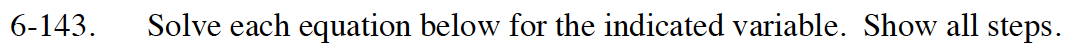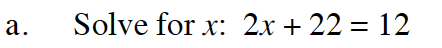x = −5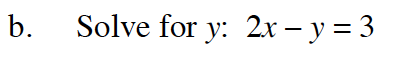Add y to both sides of the equation.

How can you move 3 to isolate y on the right side of the equation?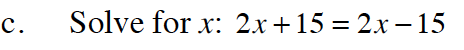If you subtract 2x from both sides of the equation, what kind of statement is left? Is there any value of x that will make this equation true?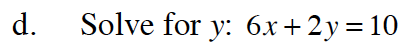y = 5 − 3x